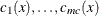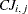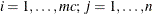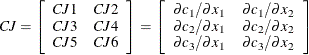### JACNLC Statement

JACNLC variables ;

The JACNLC statement defines the Jacobian matrix for the system of constraint functions. The statements list thevariable names which correspond to the elements,, of the Jacobian matrix by rows.

For example, the statements

      nlincon c1-c3;
decvar  x1-x2;
jacnlc  cj1-cj6;


correspond to the Jacobian matrixTherows of the Jacobian matrix must be in the same order as thecorresponding names of nonlinear constraints listed in the NLINCON statement. Thecolumns of the Jacobian matrix must be in the same order as thecorresponding parameter names listed in the DECVAR statement. To specify the values of nonzero derivatives, the variables specified in the JACNLC statement must be defined on the left-hand side of algebraic expressions in programming statements.

For example,

   array cd[3,4] cd1-cd12;
nlincon c1-c3 >= 0;
jacnlc cd1-cd12;

c1 = 8 - x1 * x1 - x2 * x2 - x3 * x3 - x4 * x4 -
x1 + x2 - x3 + x4;
c2 = 10 - x1 * x1 - 2 * x2 * x2 - x3 * x3 - 2 * x4 * x4 +
x1 + x4;
c3 = 5 - 2 * x1 * x2 - x2 * x2 - x3 * x3 - 2 * x1 + x2 + x4;

cd[1,1]= -1 - 2 * x1;   cd[1,2]= 1 - 2 * x2;
cd[1,3]= -1 - 2 * x3;   cd[1,4]= 1 - 2 * x4;
cd[2,1]=  1 - 2 * x1;   cd[2,2]= -4 * x2;
cd[2,3]= -2 * x3;       cd[2,4]= 1 - 4 * x4;
cd[3,1]= -2 - 4 * x1;   cd[3,2]= 1 - 2 * x2;
cd[3,3]= -2 * x3;       cd[3,4]= 1;